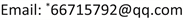﻿ 对分数运算及其相关内容的认识和思考——以人教版与北师版教材对比为例 Understanding and Thinking about Fractional Arithmetic and Its Related Contents—Taking the Comparison of Textbooks between PEP and BNUP as an Example

Vol. 11  No. 04 ( 2021 ), Article ID: 43887 , 6 pages
10.12677/AE.2021.114189

——以人教版与北师版教材对比为例Understanding and Thinking about Fractional Arithmetic and Its Related Contents

—Taking the Comparison of Textbooks between PEP and BNUP as an Example

Zhen Tang, Lin Chen*

College of Mathematics and Statistics, Yili Normal University, Yining XinjiangReceived: Jun. 12th, 2021; accepted: Jul. 9th, 2021; published: Jul. 15th, 2021ABSTRACT

Fractional arithmetic is the key and difficult part of “Number and Algebra” in primary school mathematics, and it is also an important expansion of the concept of number. This paper adopts content analysis method and literature research method, taking textbooks of PEP and BNUP as examples, conducts a comparative study on the content related to fractional arithmetic from many aspects, and the results provide some theoretical reference for further textbook reform in the future.

Keywords:Fractional Arithmetic, PEP, BNUP, The Comparison of Textbooks1. 引言

2. 研究方法及对象

2.1. 研究方法

2.2. 研究对象

3. 研究结果

3.1. 内容结构对比Table 1. PEP edition primary school mathematics textbook (PEP)

Table 2. Beijing Normal University version of elementary school mathematics textbook (BNUP)

3.2. 引入对比

3.3. 总结

“新疆高校科研计划重点项目”(XJEDU2016I043)；“伊犁师范大学博士科研启动基金项目”(2017YSBS08)。

Understanding and Thinking about Fractional Arithmetic and Its Related Contents—Taking the Comparison of Textbooks between PEP and BNUP as an Example[J]. 教育进展, 2021, 11(04): 1228-1233. https://doi.org/10.12677/AE.2021.114189

1. 1. 中华人民共和国教育部. 义务教育数学课程标准(2011年版) [M]. 北京: 北京师范大学出版社, 2012.

2. 2. 马鹏. 小学数学课程标准与教材研究[M]. 北京: 高等教育出版社, 2016.

3. NOTES

*通讯作者。